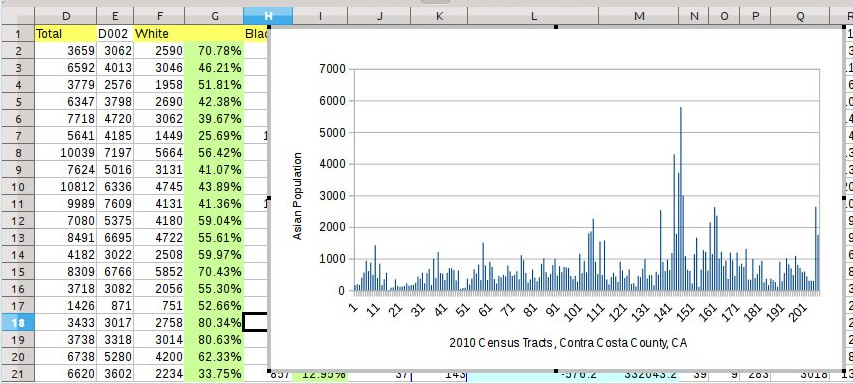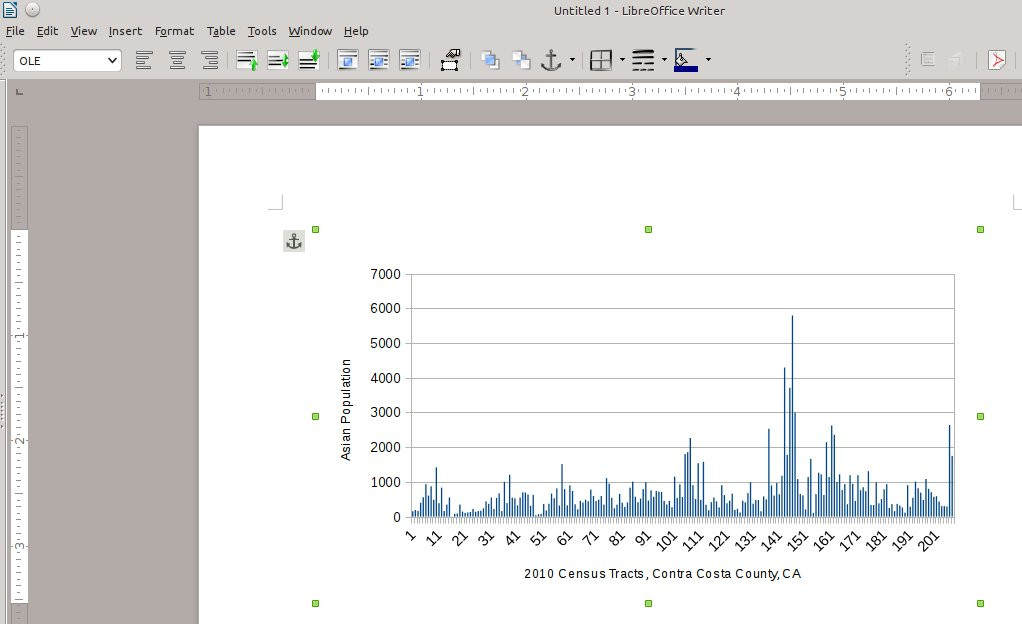A lot of quantitative analysis can be done using spreadsheets. In this assignment you will do a series of operations using spreadsheets, to give you a sense of what is possible, and to review some statistical concepts along the way. Part of the advantage of spreadsheets is that this kind of software is widely available. One version is Google Sheets, which you can access through your browser (there may even be a version that you can use offline at this point). Another version Microsoft Excel, which is a standard part of MS Office. A third version is LibreOffice Calc. It is free, and it runs a little more cleanly than MS Excel, but you will have to download and install it.

Open up the DEC_10_SF1_P5.csv file in a spreadsheet program. Save As… a different file. You pick the name, but make sure to save it in a spreadsheet format: .xlsx or .ods, not .csv.

As mentioned in class, you have to be careful how you import data into a spreadsheet, because it will start auto-formatting numbers. When using census tables for California, that is a problem because the “code number” for California is 06, and that means the short version of the geocode for your census-tracts will look something like 06013301000 (California=06; Contra Costa=013; 3010000=Census tract 3010.00). As I have shown in class, LibreOffice Calc will allow you to specify whether it should treat that number-string as calculable numbers (in which case it will strip off the leading zero and corrupt that data), or treat it as text-characters.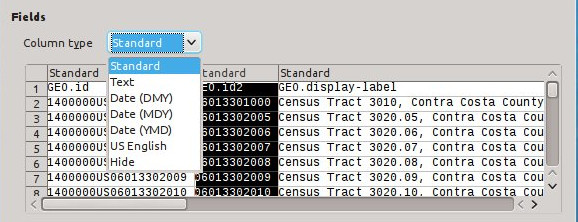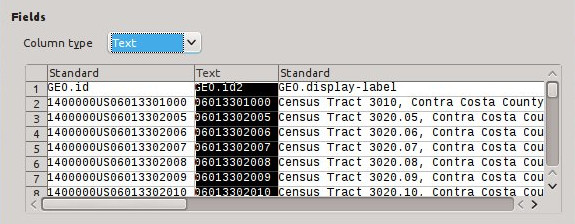In Excel, you have to go through a few more steps to signal to the software that it should import that column as text. I wrote a tutorial which explains the procedure in Excel here: AFF_CensusData_for_GIS_140510.

### Viewing the data, and basic analysis

Once you have some census data open in your spreadsheet, a first level of analysis is to just look at the raw numbers. Tables P1 and P5 are usually pretty big, with more than a dozen columns (variables) and more than 100 records (in rows). So you will not be able to easily absorb and describe the patterns of so much raw-number data, but if you just look at a few lines, you should see some patterns.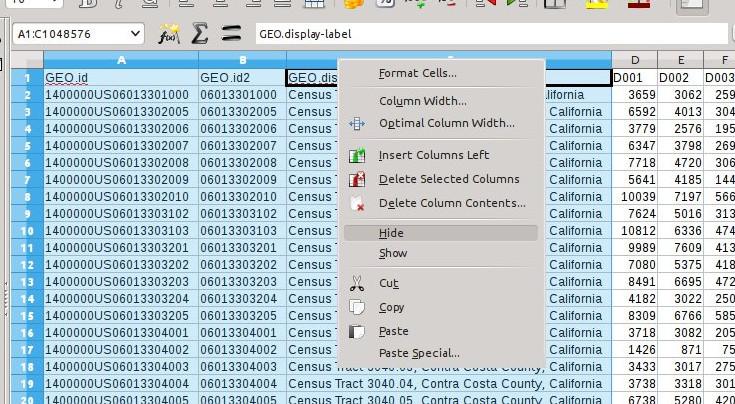If you are going to view data in a spreadsheet, it helps to control your view. In this screenshot, I have selected the firs three columns and I am about to Hide them.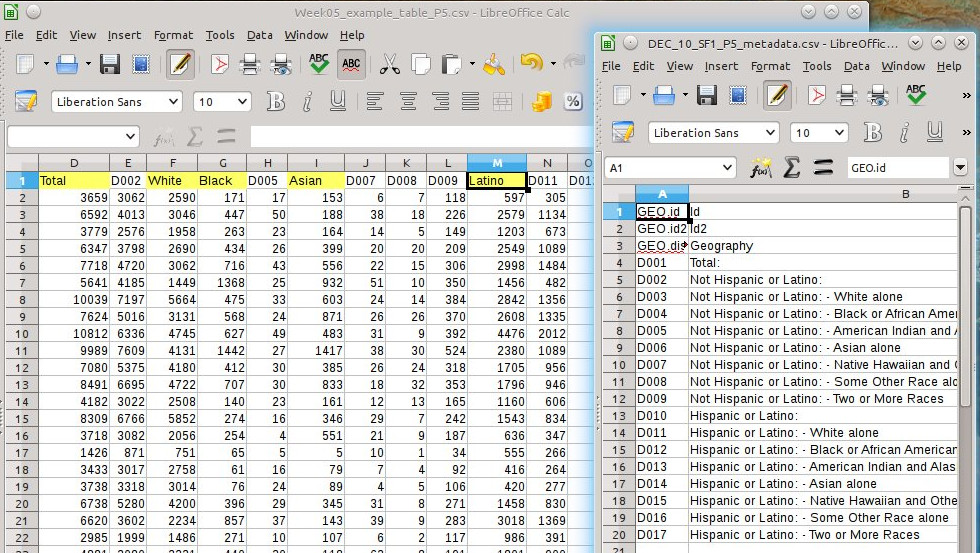Metadata file (on right) helps me rename column-labels to something intelligible.

Open up the DEC_10_SF1_P5_metadata.csv for your county to find out what the column (variable) names mean in the main file. Back in the main data file, rename D001 to Total; rename D003 to White; rename D004 to Black; rename D006 to Asian; rename D010 to Latino. These are the four largest groups within California, so I encourage you to get used to these particular codes and I encourage you to focus your term-paper analysis on these groups. California is a much more complex, plural society that many parts of America, so we need to go beyond Massey & Denton’s Black/White analysis in American Apartheid.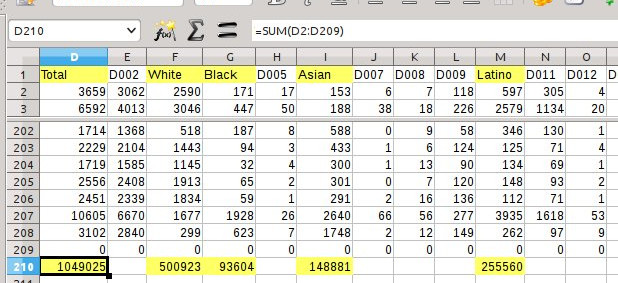Here is another tip about view-control: you can split the view so that you can see the top and the bottom of the data-set at the same time. Note that the heavy box signifies the selected cell, and the formula within that cell is displayed up in the Formula Input Bar.

At the bottom of each of these five columns, sum the value. As we discussed in class, if you find a Tract with no population, you should omit it from the calculations. In this spreadsheet, you could delete that row if you want, but I prefer to keep it and just make sure I don’t include it in the cell-range of the calculations.

You can see the formula-syntax up in the Formula Input Bar. The equals sign at the beginning signals that this will be an equation. The SUM is the actual function. The coordinates of the first and last cells in the range are given as column-letter followed by row-number, with no space. The fact that it is a range of cells is marked by a colon.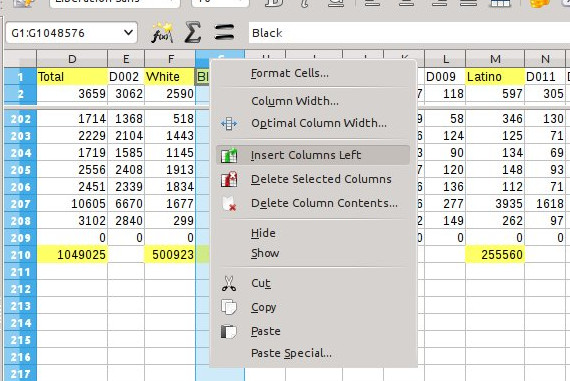Here I am about to insert a new column to the left of Black, then I will insert another to the right of Black…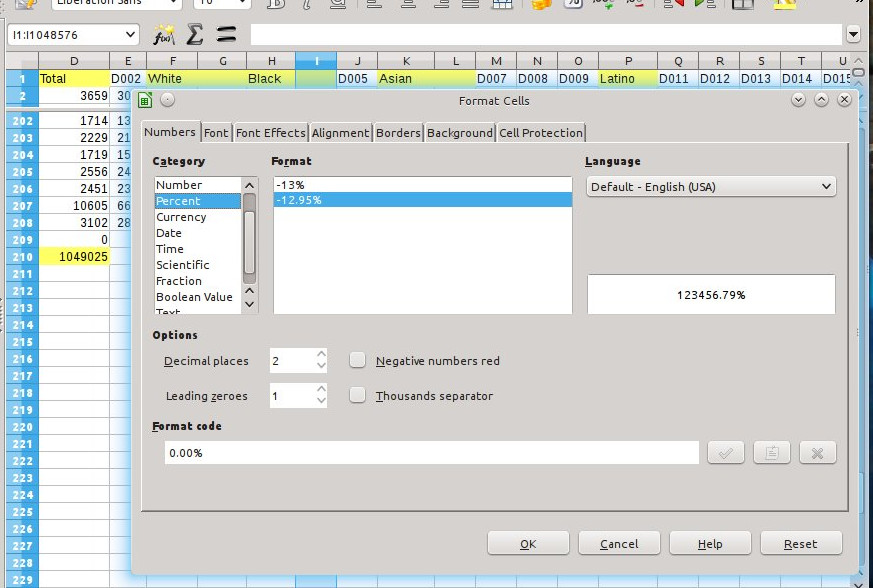This is the Format Cells dialog. Under the other tabs you can change the font size and alignment, but on the first tab you can define the data-format of each cell.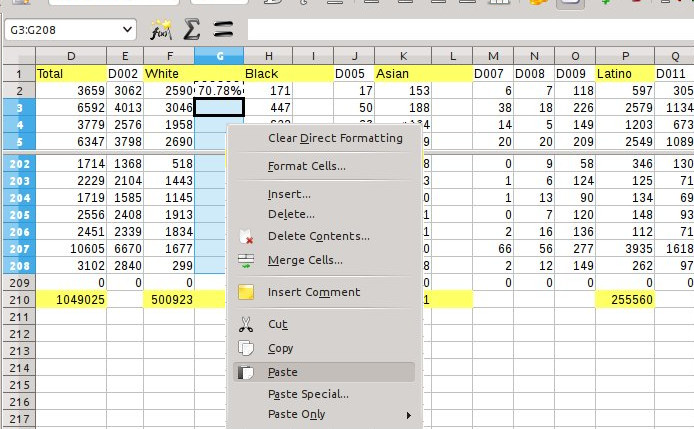Up in the first data-cell in the column (row 2), I just entered the formula. Then I copied that cell. Then I selected all the other cells below it in the column. Now I am about to paste the formula into those cells.

In this case, the formula syntax is fairly simple:
=[number of African-Americans in tract] divided by [total number in tract].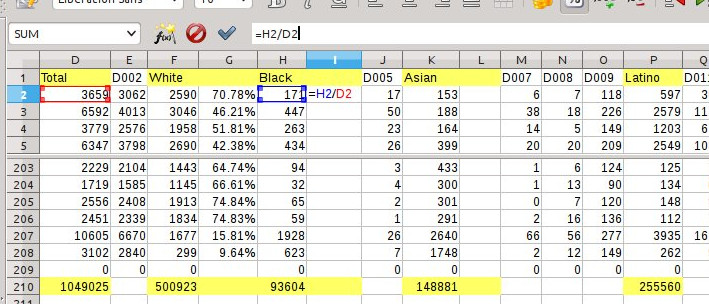Here I have selected the Formula Input Field itself. Now the relevant cells are called out in colors.

Now I can copy cell I2, then highlight cells I3:I208, and paste the equation into that whole cell-range. The software will dynamically adapt the cell-coordinates, in this case by adjusting the row-numbers within the equation to match the row-number in which the equation is being pasted.

Visually compare these results. Some tracts will have much higher and lower proportions of Whites. Likewise for Blacks. They are not necessarily inversely related, because the largest single group in California is actually Hispanics (of all races), and in some tracts the proportion of Asian-Americans is also very high. Highlight the highest proportions for Black and Whites with a green cell-color; highlight the lowest proportion with an orange cell-color. Save your work.

### Calculating the standard deviation in a step-wise way

On Monday, 9/22 I showed that we can start calculating the standard deviation of a set of data, through a series of calculations in the spreadsheet. For this assignment, calculate the standard deviation of the number of Asian-Americans in the census-tracts of your county. The purpose of this exercise is to get you used to doing a series of spreadsheet operations, and to understand the relationship between standard deviation and the data that it describes.

The first step in calculating the standard deviation is to determine the mean value of the data. In this case, it is the arithmetic average, which is the sum of the values in all the cases divided by the number of cases. In this dataset, the number of cases (N) =207, since we omit the first row and in this case the Tract shown on row 209 has no population. In the screenshot below, the mean is shown in cell K211.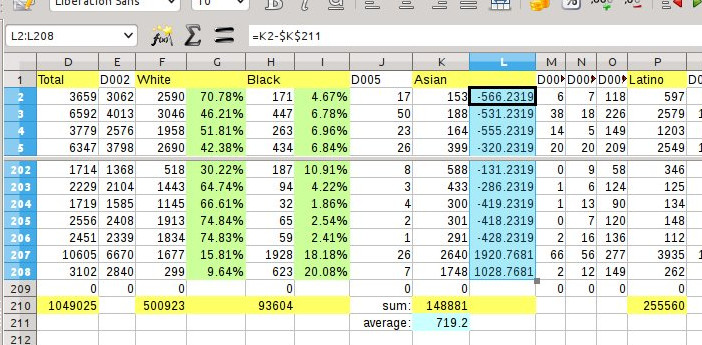Another note about visibility: I am tinting the cells in different colors as I proceed with these operations so that you can track each step.

In Column L, I have taken the second step in the calculation: finding the difference between each value in the dataset and the mean-value of the set. Many tracts are well below the mean, and some are well above the mean, yielding strongly positive and negative results that would almost cancel each other out if I summed up Column L.

To correct for this in the old-school 20th century way, we square the differences so that all the results become positive (and unfortunately huge). The standard deviation has been used as a statistical measure for decades. Using computers, we could use different methods of calculation that were too labor-intensive to do by hand in the past. But since we want to compare our data to previous research, we use this ‘legacy’ method of calculation.

Note the syntax for the formula: I wanted to square the value in cell L2. The carat symbol (SHIFT + 6) represents exponents in spreadsheet formula syntax.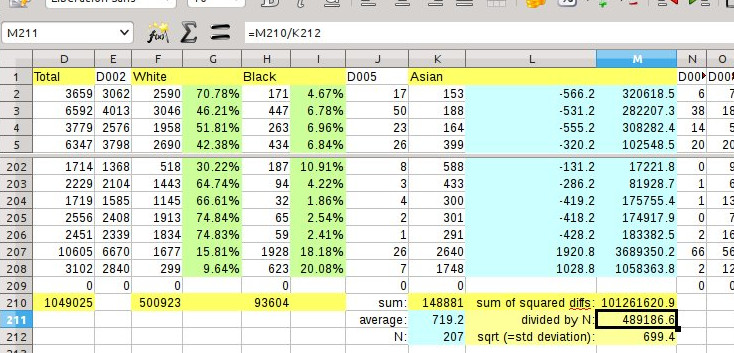Sum of squares divided by the number of cases (N). Hey! Was there a Swede in here doing the captions?

The sum-of-squares for a large dataset would be massive, whereas for a smaller dataset it would be smaller. To compare apples-to-apples we need to “normalize the data” by dividing total sum by the number of cases (N). The result is the Variance, a basic measure of dispersion that is independent of data-set size. We can compare the variance of Asian-Americans in tracts in Santa Cruz county with Los Angeles county, even though LA county has maybe 50x the number of tracts (and absolute population). Variance–the degree of dispersion away from the mean–could be high or low for counties, regardless of their size. So variance is a useful number for comparisons between related datasets. But since it still represents the square-of-difference, it does not easily relate to the raw numbers within the dataset. So…

The square root of the variation yields a number which is an expression of the ‘standard’ degree of variation of data-points above and below the mean. So we could stop here. The manual-calculation of standard deviation is done.

But that standard deviation number raises a red flag. It means that the ‘typical’ variation of Asian-American tract-populations above and below 719 persons is +/- 699 persons! That is REALLY dispersed! So now I am going to build a histogram of the Asian-American tract-populations to see what the data-distribution looks like.

### A graphic check on really high dispersal

So let us build a histogram. Select the data-range you want to include in the chart. Then click the ‘Chart’ icon (or, in LibreOffice Calc, go to Insert -> Object -> Chart…) to invoke the Chart Wizard.

The histogram is a type of “Column” chart.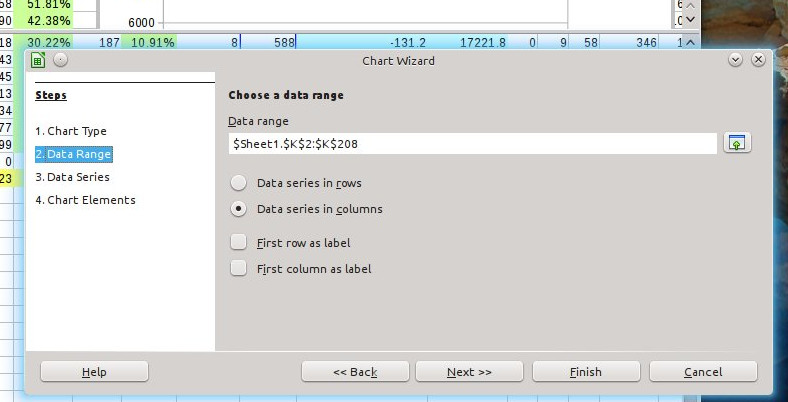In this step you can verify and revise the data-ranges you are using for the chart.